# RS Aggarwal Class 7 Solutions Chapter 10 Percentage Ex 10C

## RS Aggarwal Class 7 Solutions Chapter 10 Percentage Ex 10C

These Solutions are part of RS Aggarwal Solutions Class 7. Here we have given RS Aggarwal Solutions Class 7 Chapter 10 Percentage Ex 10C.

Other Exercises

Objective Questions :
Mark (✓) against the correct answer in each of the following :
Question 1.
Solution:
(b) $$\frac { 3 }{ 4 }$$ = $$\frac { 3 }{ 4 }$$ x 100 = 75 %

Question 2.
Solution:
(c)
2 : 5 = $$\frac { 2 }{ 5 }$$ = $$\frac { 2 }{ 5 }$$ x 100 = 40%

Question 3.
Solution:
(c)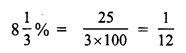Question 4.
Solution:
(c) x% of 75 = 9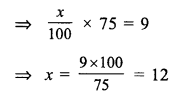Question 5.
Solution:
(d)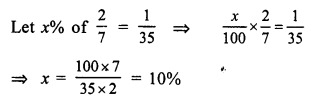Question 6.
Solution:
(b)
Let x% of 1 day = 36 minutes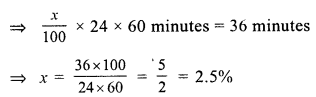Question 7.
Solution:
(a)
Let x be the required number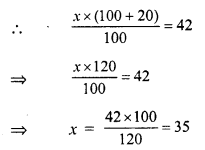Question 8.
Solution:
(b)
Let x be the required number, then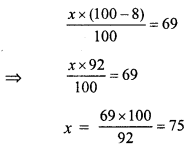Question 9.
Solution:
(d)
Let ore = x, then
5% of x = 400g
$$\frac { x x 5 }{ 100 }$$ 400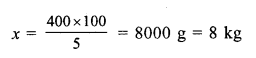Question 10.
Solution:
(b)
Let gross value of T.V = x
Commission = 10%
After deducting commission, the value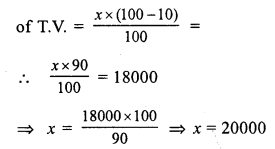Question 11.
Solution:
(b)
Increase in salary = 25%
Let original salary = x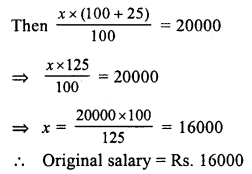Question 12.
Solution:
(c)
Let x be the number of total examinees
No. of examinees passed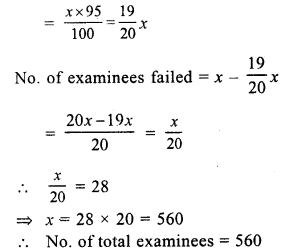Question 13.
Solution:
(c)
Let total number of apples = x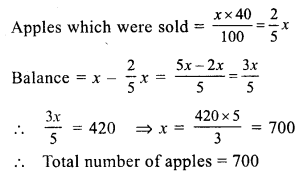Question 14.
Solution:
(b)
Present value of machine = Rs. 25000
Rate of depreciation = 10% p.a.
Value of machine after one year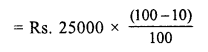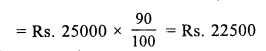Question 15.
Solution:
(c) Let x be numbers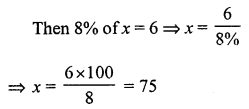Question 16.
Solution:
(c) 60% of 450
= $$\frac { 60 }{ 100 }$$ x 450 = 270

Question 17.
Solution:
(d) Rate of reduction = 6%
Price after reduction = Rs. 658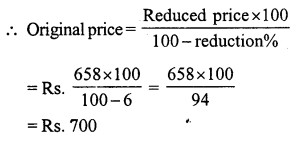Question 18.
Solution:
(b) Boys = 70% of students
No. of girls = 240
Girls percentage = 100 – 70 = 30%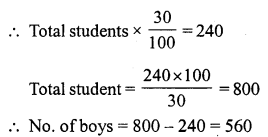Question 19.
Solution:
(c) Let number = x
11% of x – 7% of x = 18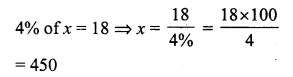Question 20.
Solution:
(a) Let number = x
35% of x + 39 = x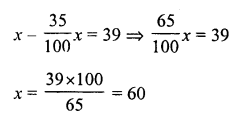Question 21.
Solution:
(c) Pass marks = 36%
A students get =145 marks
But failed by 3 5 marks
Then pass marks = 145 + 35 = 180
Maximum marks = $$\frac { 180 x 100 }{ 36 }$$ = 500

Question 22.
Solution:
(d) Let number be = x
Then decreasing by 40%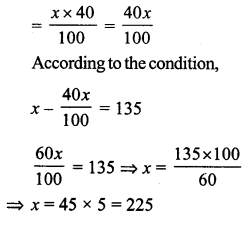Hope given RS Aggarwal Solutions Class 7 Chapter 10 Percentage Ex 10C are helpful to complete your math homework.

If you have any doubts, please comment below. Learn Insta try to provide online math tutoring for you.